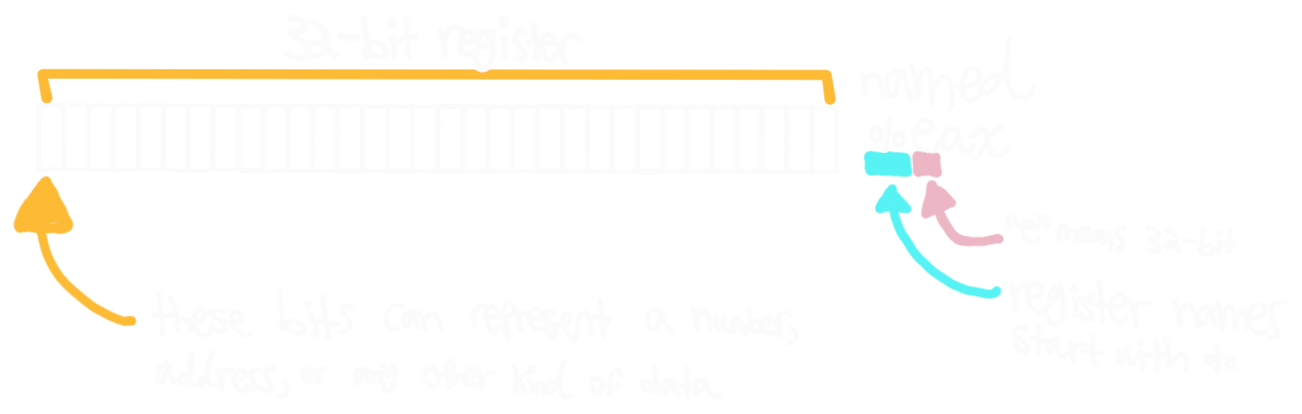hello

# Types of Data

## Immediates

Immediates are constants. There are several notations for them, but they consistently start with a dollar sign:

• Decimal Constants: $11 = $\left(11\right)_{10}$ • Hex Constants: $0x11 = $\left(11\right)_{16} = \left(17\right)_{10}$
• String Constants: $str ## Registers Registers are variables of a fixed-width. For example:Unlike variables, there are only so many registers, and we cannot “declare” more. They must be used judiciously. ## Memory # mov and retq ten.c int ten() { return 10; }  $\leftrightarrow$ clang -S ten.c ten: mov$10, %eax
retq


## mov Instruction

x86-64 [data to register]

mov $imm, %dst mov %src, %dst mov (%src), %dst  x86-64 [register to memory] mov %src, (%dst) mov$imm, (%dst)


x86-64 [memory displacement]

mov D(%src), %dst
mov %src, D(%dst)


$\leftrightarrow$

C Pseudocode

dst = imm
dst = src
dst = *src


C Pseudocode

*dst = src
*dst = imm


C Pseudocode

dst = *(src + D)
*(dst + D) = src


There is no instruction to mov directly from memory to memory!

D is an immediate without the \$ prefix.

## ret Instruction

x86-64

retq


$\leftrightarrow$

C Pseudocode

return %eax


Notice that %eax always contains the return value.

We cannot return any other register!

# Identity Function (Arguments)

identity.c

int identity(int x) {
return x;
}


$\leftrightarrow$

clang -S identity.c

identity:
mov  %edi, %eax
retq